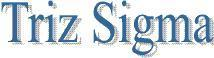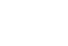Process Capability Introduction The capability of a process is some measure of the proportion of in-specification items the process produces when it is in a state of statistical control. Process capability addresses the natural variation of a process or operation. If we lived in a perfect world and there was no variation, there would not be any quality problems assuming the process was centered within the specification limits. According to Juran, process capability is the measured, inherent reproducibility of the product turned out by a process. It is worth considering each part of this definition briefly. Calculation of the Process Capability You can tell a lot about your process by using histograms and control charts together. It is also a widely-accepted practice to express process capability using the following indices: Process Capability Ratio (Cp): Cp is the simple process capability index. The Capability Index (Cp), as defined by the equation: Cp = (USL - LSL)/6*sigma where Cp = Capability index, USL - LSL = Upper Specification limit - Lower Specification Limit, 6*sigma = process capability Three possible outcomes derive from this calculation. These are sometimes referred to following cases A Cp of < 1 suggests that the numerator is smaller than the denominator. Thus, we are exceeding the space allowed by the tolerance. This is an unacceptable situation. A Cp of exactly 1 indicates that we are using up precisely the amount of tolerance space provided by the specification. If the process drifts even a little bit assuming it was centered in the first place, there will be a problem with defects. A Cp of > 1 is what is desirable with infinity being the largest figure this index can assume. This of course is impossible since it would mean that we are dividing by 0 and making each part exactly the same As a practical matter, major automakers, for example, are calling for Cps of 1.33 and more to assure themselves that suppliers are capable of providing high quality parts. This implies that suppliers are operating at 4 sigma and more. The Cp index indicates whether the process variation is acceptable, that is, if the process were centered correctly, could it produce all good product. For this reason it is a valuable in-plant tool, which can help to pinpoint whether excessive variation is responsible for the problem, or not. It does not measure whether or not the process is centered properly. Therefore, it does not provide protection against out of specification product which results from poor centering.Process Capability Ratio (Cpk):Bear in mind that specification limits are not statistically determined, but rather are set by customer requirements and process economics. For this reason, another measure called Cpk is usually of primary interest for customers. The Cpk index measures the effect of both center and variation at the same time. Thus, Cpk is calculated by finding the lesser of: Cpk = (USL  mean) / 3*sigma or = (LSL  mean) / 3*sigma The interpretation of Cpk is as follows: If the Cpk is negative, then the process center is aimed outside of the specification limits and a high percentage of out of specification product can be expected. Cpk < 1.00 - Unacceptable process/Part of process distribution out of spec 1.00 < Cpk < 1.33 -Marginal process /Process distribution barely within spec Cpk > 1.33- Acceptable process/Process distribution well within spec The Cpk can never be higher than the Cp value for a process. If the process is centered exactly in the middle of the specification, the two values will be equal. If the Cp is good but the Cpk is poor, the problem can be corrected by changing the center. When both Cp and Cpk are poor, process variation must be reduced.